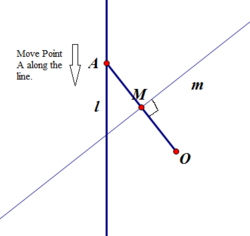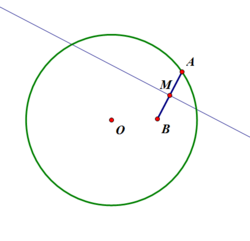# Conics – A graphic explanation with GIFsA conic section is the intersection of a plane and a double right circular cone. By changing the angle and location of the intersection, we can produce different types of conics. Traditionally, the three types of conic section are the hyperbola, the parabola, and the ellipse. The circle is a special case of the ellipse, and is sometimes called the fourth type of conic section. A conic consists of those points whose distances to some point, called a focus, and some line, called a directrix, are in a fixed ratio, called the eccentricity.Image Courtesy: mathdemos.org

The type of a conic corresponds to its eccentricity, those with eccentricity less than 1 being ellipses, those with eccentricity equal to 1 being parabolas, and those with eccentricity greater than 1 being hyperbolas. In the focus-directrix definition of a conic the circle is a limiting case with eccentricity 0. In modern geometry certain degenerate cases, such as the union of two lines, are included as conics as well.

## ParabolaImage Courtesy: thevirally.com

A two-dimensional, mirror-symmetrical conic curve, a parabola is approximately U-shaped when oriented as shown in the animation, but which can be in any orientation in its plane. It fits any of several superficially different mathematical descriptions which can all be proved to define curves of exactly the same shape.

### Parabola EnvelopeImage Courtesy: mathforum.org

In the above animation, line m is the sweeping line and is perpendicular to segment OA at its midpoint M. Since point O is fixed and if we slide along point A along line l, line m will sweep out a parabola with O as its focus and l as its directrix.

### Reflecting Property of ParabolaImage Courtesy: westmont.edu

The above animation illustrates the reflecting property of parabolas. This mean that if any object (such as a light wave, sound wave, or rubber ball) is projected in a straight line from the focus, it will be reflected by the parabola in a direction parallel to the axis of symmetry (which is the x-axis in this case). The mathematical proof of this property can also be seen.

## EllipseImage Courtesy: thevirally.com

An ellipse is a curve on a plane surrounding two focal points such that a straight line drawn from one of the focal points to any point on the curve and then back to the other focal point has the same length for every point on the curve. Ellipses are the closed type of conic section: a plane curve that results from the intersection of a cone by a plane. Ellipses are common in Astronomy, physics and engineering.  For example, the orbits of the planets are ellipses with the Sun at one of the focal points.  The equation of an ellipse whose major and minor axes coincide with the Cartesian axes is:

x2/a2 + y2/b2 =1

### Ellipse EnvelopeImage Courtesy: mathforum.org

The envelope of an ellipse is illustrated in this animation. The sweeping line, which is the perpendicular bisector of line AB now marks the boundary of an ellipse. The point A slides on a circle which results in an ellipse with O and B as its foci.

### Parametric form in Canonical positionImage Courtesy: en.wikipedia.org

The animation shows the parametric equation for the ellipse (red) in canonical position. The eccentric anomaly t is the angle of the blue line with the X-axis. For an ellipse in canonical position (center at origin, major axis along the X-axis), the equation simplifies to

X (t) = a cos t

Y (t) = b sin t

### Ellipse as HypotrochoidImage Courtesy: wikimedia.org

The ellipse is a special case of the hypotrochoid when R = 2r.

### Reflecting property of EllipsesImage Courtesy: westmont.edu

Consider an arbitrary (but fixed) positive number d, and two fixed points (called foci) whose distance between them is less than d. An ellipse is the collection of points satisfying the following property: for any given point in this collection, the sum of the distances between this given point and the two foci equals d. The above animation illustrates the reflecting property of ellipses, viz., that if any object (such as a light wave, sound wave, or rubber ball) is projected in a straight line from one focus, it will be reflected by the ellipse in such a way that it will pass through the other focus.

## HyperbolaImage Courtesy: thevirally.com

A hyperbola is an open curve with two branches, the intersection of a plane with both halves of a double cone. If the plane intersects both halves of the double cone but does not pass through the apex of the cones then the conic is a hyperbola. The plane does not have to be parallel to the axis of the cone; the hyperbola will be symmetrical in any case. Hyperbolas arise in many ways: as the curve representing the function f(x) = 1/x in the Cartesian plane, as the appearance of a circle viewed from within it, as the path followed by the shadow of the tip of a sundial and as the shape of an open orbit (as distinct from a closed elliptical orbit).

### Reflection property of HyperbolaImage Courtesy: cda.morris.umn.edu

The animations show the hyperbola in blue lines with red dots being its foci. Any particle that is directed towards a focus of a hyperbola will be reflected towards the other focus. This reflection property makes hyperbolas useful in telescopes, where a hyperbola shaped mirror can be used to direct light towards a point.

### Hyperbola EnvelopeImage Courtesy: mathforum.org

The animation shows the enveloping process of a hyperbola. In this animation, the sweeping line is perpendicular to the segment AB. Point B lies outside the circle and the sweeping line results in a hyperbola with O and B as its foci.

### HyperboloidImage Courtesy: eng.buffalo.edu

The animation shows the hyperboloid of a single sheet. In mathematics, a hyperboloid is a quadric type of surface in three dimensions and is described by the equation

x2/a2 + y2/b2 – z2/c2 = 1

24055   27/08/2014